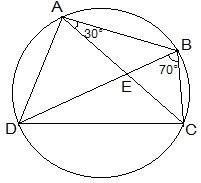# ABCD is a cyclic quadrilateral whose diagonals intersect at a point E. If ∠ DBC = 70°, ∠ BAC is 30°, find ∠ BCD. Further, if AB = BC, find ∠ ECD.#### Solution

Angles in the same segment are equal.

So, ∠ CBD = ∠ CAD

∠ BAD will be equal to the sum of angles BAC and CAD.

= 30°+70°

We know that the opposite angles of a cyclic quadrilateral sums up to 180 degrees.

So,

We know that ∠ BAD = 100°

So, ∠ BCD = 80°

Consider the ΔABC.

Given that AB = BC

Also, ∠ BCA = ∠ CAB (They are the angles opposite to equal sides of a triangle)

∠ BCA = 30°

also, ∠ BCD = 80°

∠ BCA +∠ ACD = 80°

Thus, ∠ ACD = 50° and ∠ ECD = 50°